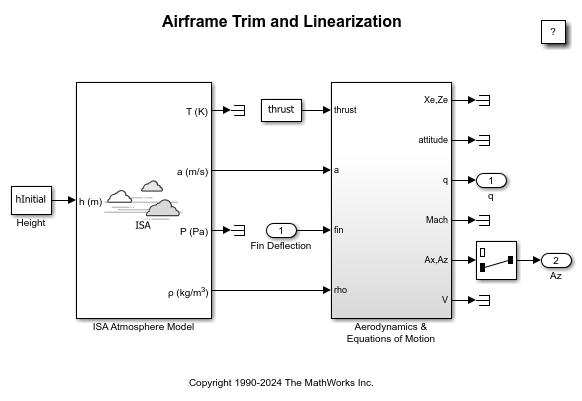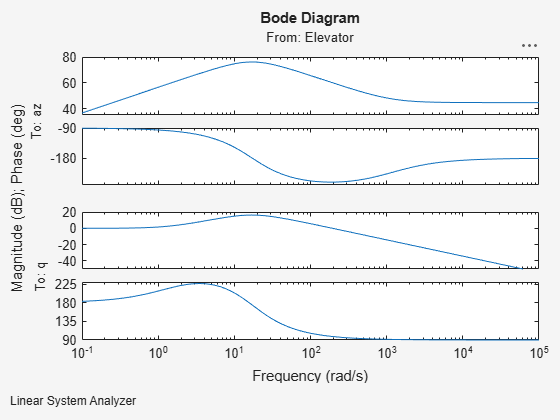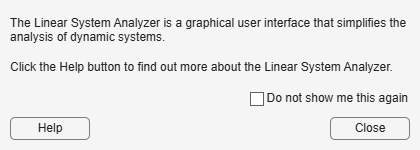# Airframe Trim and Linearize

This example shows how to trim and linearize an airframe using Simulink® Control Design™.

Designing an autopilot using classical design techniques requires linear models of the airframe pitch dynamics for a number of trimmed flight conditions. MATLAB® can determine the trim conditions and derive linear state-space models directly from the nonlinear Simulink® and Aerospace Blockset™ model. This saves time and helps to validate the model. The functions provided by Simulink Control Design allow you to visualize the behavior of the airframe in terms of open-loop frequency (or time) responses.

### Initialize Guidance Model

The first problem is to find the elevator deflection, and the resulting trimmed body rate (q), which will generate a given incidence value when the missile is traveling at a set speed. Once the trim condition is found, a linear model can be derived for the dynamics of the perturbations in the states around the trim condition.

```open_system('aero_guidance_airframe'); ```### Define State Values

```h_ini = 10000/m2ft; % Trim Height [m] M_ini = 3; % Trim Mach Number alpha_ini = -10*d2r; % Trim Incidence [rad] theta_ini = 0*d2r; % Trim Flightpath Angle [rad] v_ini = M_ini*(340+(295-340)*h_ini/11000); % Total Velocity [m/s] q_ini = 0; % Initial pitch Body Rate [rad/sec] ```

### Set Operating Point and State Specifications

The first state specifications are Position states, the second state specification is Theta. Both are known, but not at steady state. The third state specifications are body axis angular rates of which the variable w is at steady state.

```opspec = operspec('aero_guidance_airframe'); opspec.States(1).Known = [1;1]; opspec.States(1).SteadyState = [0;0]; opspec.States(2).Known = 1; opspec.States(2).SteadyState = 0; opspec.States(3).Known = [1 1]; opspec.States(3).SteadyState = [0 1]; ```

### Search for Operating Point, Set I/O, then Linearize

```op = findop('aero_guidance_airframe',opspec); io(1) = linio('aero_guidance_airframe/Fin Deflection',1,'input'); io(2) = linio('aero_guidance_airframe/Selector',1,'output'); io(3) = linio(sprintf(['aero_guidance_airframe/Aerodynamics &\n', ... 'Equations of Motion']),3,'output'); sys = linearize('aero_guidance_airframe',op,io); ```
``` Operating point search report: --------------------------------- opreport = Operating point search report for the Model aero_guidance_airframe. (Time-Varying Components Evaluated at time t=0) Operating point specifications were successfully met. States: ---------- Min x Max dxMin dx dxMax __________ __________ __________ __________ __________ __________ (1.) aero_guidance_airframe/Aerodynamics & Equations of Motion/ Equations of Motion (Body Axes)/Position 0 0 0 -Inf 967.6649 Inf -3047.9999 -3047.9999 -3047.9999 -Inf -170.6254 Inf (2.) aero_guidance_airframe/Aerodynamics & Equations of Motion/ Equations of Motion (Body Axes)/Theta 0 0 0 -Inf -0.21604 Inf (3.) aero_guidance_airframe/Aerodynamics & Equations of Motion/ Equations of Motion (Body Axes)/U,w 967.6649 967.6649 967.6649 -Inf -14.0977 Inf -170.6254 -170.6254 -170.6254 0 -7.439e-08 0 (4.) aero_guidance_airframe/Aerodynamics & Equations of Motion/ Equations of Motion (Body Axes)/q -Inf -0.21604 Inf 0 3.3582e-08 0 Inputs: ---------- Min u Max _______ _______ _______ (1.) aero_guidance_airframe/Fin Deflection -Inf 0.13615 Inf Outputs: ---------- Min y Max ________ ________ ________ (1.) aero_guidance_airframe/q -Inf -0.21604 Inf (2.) aero_guidance_airframe/az -Inf 199.2481 Inf ```

### Select Trimmed States, Create LTI Object, and Plot Bode Response

```airframe = ss(sys.A(3:4,3:4),sys.B(3:4,:),sys.C(:,3:4),sys.D); set(airframe,'inputname',{'Elevator'}, ... 'outputname',[{'az'} {'q'}]); ltiview('bode',airframe); bdclose('aero_guidance_airframe'); ```